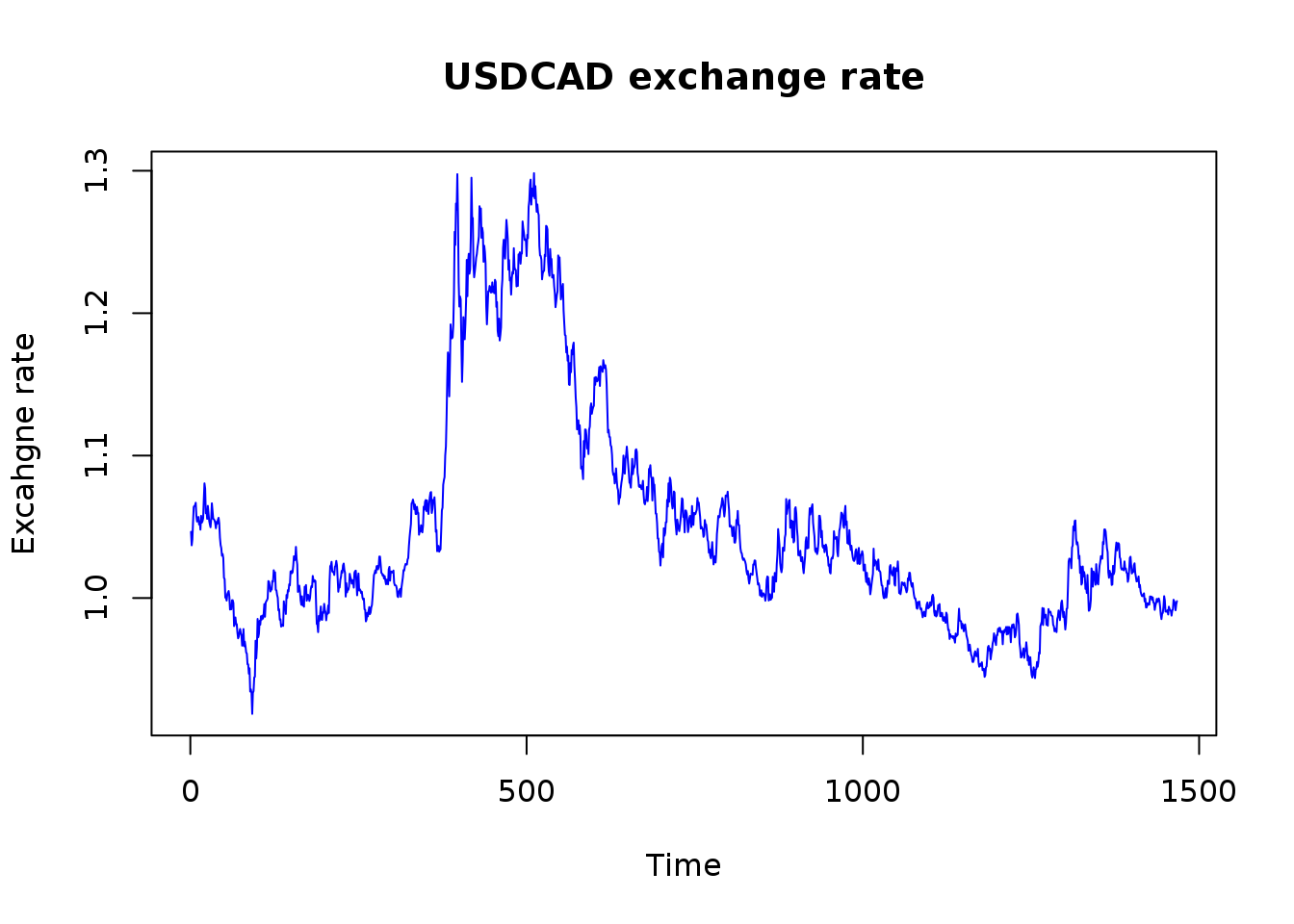# Synopsis

In this report, we analyze the mean reversion property of a pre-fabricated Forex currency pair, namely, we focus here on USDCAD, analyzing the daily close prices, from July 22, 2007 to March 28, 2012. The input data was retriever with TickDownloader. This analysis is based on the original study provided in Ernest P.Chan - Algoritmic trading winning strategies and their rationales.

# Data preprocessing

data <- read.csv("USDCAD_D1_2007_07_2012_03.csv")

# assign appropriate names to this dataset columns:
names(data) <- c("date","time","open","high","low","close","volume")

# print some of the data:
head(data)
##         date  time    open    high     low   close  volume
## 1 2007.07.23 00:00 1.04770 1.04790 1.04250 1.04650 1243279
## 2 2007.07.24 00:00 1.04660 1.04735 1.03405 1.03700 1249046
## 3 2007.07.25 00:00 1.03700 1.04480 1.03400 1.04120 1241928
## 4 2007.07.26 00:00 1.04120 1.05710 1.04029 1.05320 1295846
## 5 2007.07.27 00:00 1.05320 1.06480 1.05240 1.06390 1492336
## 6 2007.07.29 00:00 1.06401 1.06500 1.06380 1.06466   15830

We have 1467 observations available, but note that, this dataset we are only interested in the close prices for the time being, so we keep only that column:

prices <- data$close # let's now plot the data: nob <- length(prices) plot(1:nob,prices, type="l", col="blue", xlab="Time", ylab="Excahgne rate", main="USDCAD exchange rate")We see from this plot that we have roughtly the same shape as in the original study, but it also seems that we have more data points (we only have about 1200 points in the source paper). # Results ## ADF test Now we can perform the ADF test, for this we will use the tseries package, containing the adf.test function. library(tseries) # With the default number of lag coefficients: tres1 <- adf.test(prices) tres1 ## ## Augmented Dickey-Fuller Test ## ## data: prices ## Dickey-Fuller = -1.8147, Lag order = 11, p-value = 0.6568 ## alternative hypothesis: stationary # Now with only 1 lag coefficient: tres2 <- adf.test(prices,"s",k=1) tres2 ## ## Augmented Dickey-Fuller Test ## ## data: prices ## Dickey-Fuller = -2.0301, Lag order = 1, p-value = 0.5656 ## alternative hypothesis: stationary In both test cases the p.values are quite high (respectively 0.657 and 0.566) so we cannot reject the null hypothesis, and this currency is not stationary (which is of course expected). As a validation step we can recompute the ADF test result using the fUnitRoots package: library(fUnitRoots) adfTest(prices, type="ct") ## ## Title: ## Augmented Dickey-Fuller Test ## ## Test Results: ## PARAMETER: ## Lag Order: 1 ## STATISTIC: ## Dickey-Fuller: -2.0301 ## P VALUE: ## 0.5656 ## ## Description: ## Mon Mar 14 15:25:06 2016 by user: The results computed with the fUnitRoots package with type=“ct” correspond to what we computed in the tseries package. Yet, we don’t want to consider the time trend in the regression, so we should recompute the Dickey-Fuller statistic with only the constant component (eg. type = “c”): ares1 <- adfTest(prices, type="c") ares1 ## ## Title: ## Augmented Dickey-Fuller Test ## ## Test Results: ## PARAMETER: ## Lag Order: 1 ## STATISTIC: ## Dickey-Fuller: -1.8196 ## P VALUE: ## 0.3806 ## ## Description: ## Mon Mar 14 15:25:06 2016 by user: We note here that we still cannot reject the null hypothesis, since our p-value is of about 0.3806. ## Hust exponent computation We use the pracma package to compute the Hurst exponent. Note that we compute the Hurst exponent for the log prices serie. library(pracma) hres1 <- hurstexp(log(prices)) ## Simple R/S Hurst estimation: 0.8602589 ## Corrected R over S Hurst exponent: 1.000402 ## Empirical Hurst exponent: 1.227837 ## Corrected empirical Hurst exponent: 1.220362 ## Theoretical Hurst exponent: 0.522386 The most “interesting value” in the previous list is the Theoretical Hurst exponent : 0.5224. Given this value is would seem that the currency pair analyzed in this period exibited a small trending tendancy. ## Variance ratio test To check the statistical significance of this Hurst exponent value, we perform a variance ratio test. We will use the vrtest package to acheive this: library(vrtest) nob <- length(prices) lret <- log(prices[2:nob]) - log(prices[1:(nob-1)]) lres1 <- Lo.Mac(lret, kvec = 2) lres1 ##$Stats
##             M1         M2
## k=2 -0.5099503 -0.3662215

The previous statistics indicate that we have 51.00% chances that the return values follow a random walk (??? Not really sure what those numbers mean in fact), so we cannot discard this hypothesis.

As described from this page we can compute the p-value of this statistic usign the Boot.test function:

Boot.test(lret, kvec=c(2,5), nboot=500,wild="Normal")
## $Holding.Period ##  2 5 ## ##$LM.pval
##  0.72 0.90
##
## $CD.pval ##  0.896 ## ##$CI
##          2.5%    97.5%
## k=2 -1.895753 1.858923
## k=5 -1.845184 2.128662

Right now, I cannot make any sense of those results unfortunately.

## Half-life of mean reversion

To determine the half-life $$\lambda$$ of our time serie we run a regression fit with $$y(t) - y(t-1)$$ as the dependent variable and $$y(t-1)$$ as the independent variable.

nob <- length(prices)
xval <- prices[1:(nob-1)]
dy <- prices[2:nob] - prices[1:(nob-1)]
reg <- lm(dy ~ xval)

plot(xval, dy, col='blue')
abline(reg,col='red')